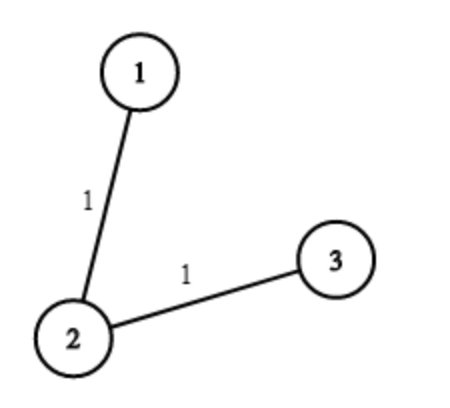# Water Supply In A Village

Posted: 2 Apr, 2021
Difficulty: Hard

## PROBLEM STATEMENT

#### For Example:

``````For ‘N’ = 3, ‘WELLS[]’ = ‘[1,2,2]’, ‘PIPES[]’ = [ [1, 2, 1], [2 , 3, 1]]. The image shows the costs of connecting houses using pipes. The best strategy is to build a well in the first house with cost 1 and connect the other houses to it with cost 2 so the total cost is 3.
``````#### Ninja wants to find out the minimum total cost to supply water to all houses in the village. Can you help the Ninja to find out this minimum cost?

##### Input Format
``````The first line of input contains an integer 'T' representing the number of test cases. Then the test cases follow.

The first line of each test case contains two integers ‘N’ and ‘K’ representing the number of Houses in the village and the size of ‘PIPES’ respectively.

The next line contains ‘N’ single space-separated integers denoting ‘WELLS[i]’.

The next ‘K’ line contains 3 single space-separated integers denoting ‘PIPES[i]’, ‘PIPES[i]’ and ‘PIPES[i]’.
``````
##### Output Format :
``````For each test case, print the minimum cost to supply water to all the houses in the village.

The output for each test case is printed in a separate line.
``````
##### Constraints :
``````1 <= T <= 100
1 <= N <= 10 ^ 2
0 <= WELLS[i] <= 10^5
1 <= K <= 10000
1 <= PIPES[i], PIPES[i] <= N
0 <= PIPES[i] <= 10^5
PIPES[i] != PIPES[i]

Where ‘T’ is the number of test cases, ‘N’ is the number of houses in the village, WELL[i]’ denotes the cost of inserting a well at house ‘i’ and ‘PIPES[i]’, ‘PIPES[i]’ and ‘PIPES’ represents the cost to connect house ‘PIPES[i]’ to ‘PIPES[i]’.

Time Limit: 1 sec
``````Approach 1

We can assume this problem as a Shortest path problem/Minimum spanning tree problem. In this problem, in a graph, consider cities as nodes, pipe connects two cities as edges with cost. Now wells cost, they are self-connected edges, we can add an extra node as root node 0, and connect all 0 and every node with costs ‘WELLS[i]’. So that we can have one graph/tree, and can get minimum spanning trees / shortest path in a graph.

Here is the complete algorithm:

1. Create a helper function ‘edgeCost(house1, house2, cost)’, where ‘cost’ is the cost to connect ‘house1’ and ‘house2’.
2. Assume ‘0’ as the root node, now build a graph with node 0 and all nodes (Houses).
3. Connect all nodes with 0 while forming the graph.
4. Turn houses into nodes well's costs and pipes' cost into edges value which connect into two cities.
5. Sort all edges (from min to max).
6. Scan all edges, check whether 2 nodes connected or not:（Union-Find method).
7. If already connected, continue to check the next edge.
8. If not yet connected, connect 2 nodes add cost to the final answer.
9. If all nodes already connected, get minimum costs, return the result.